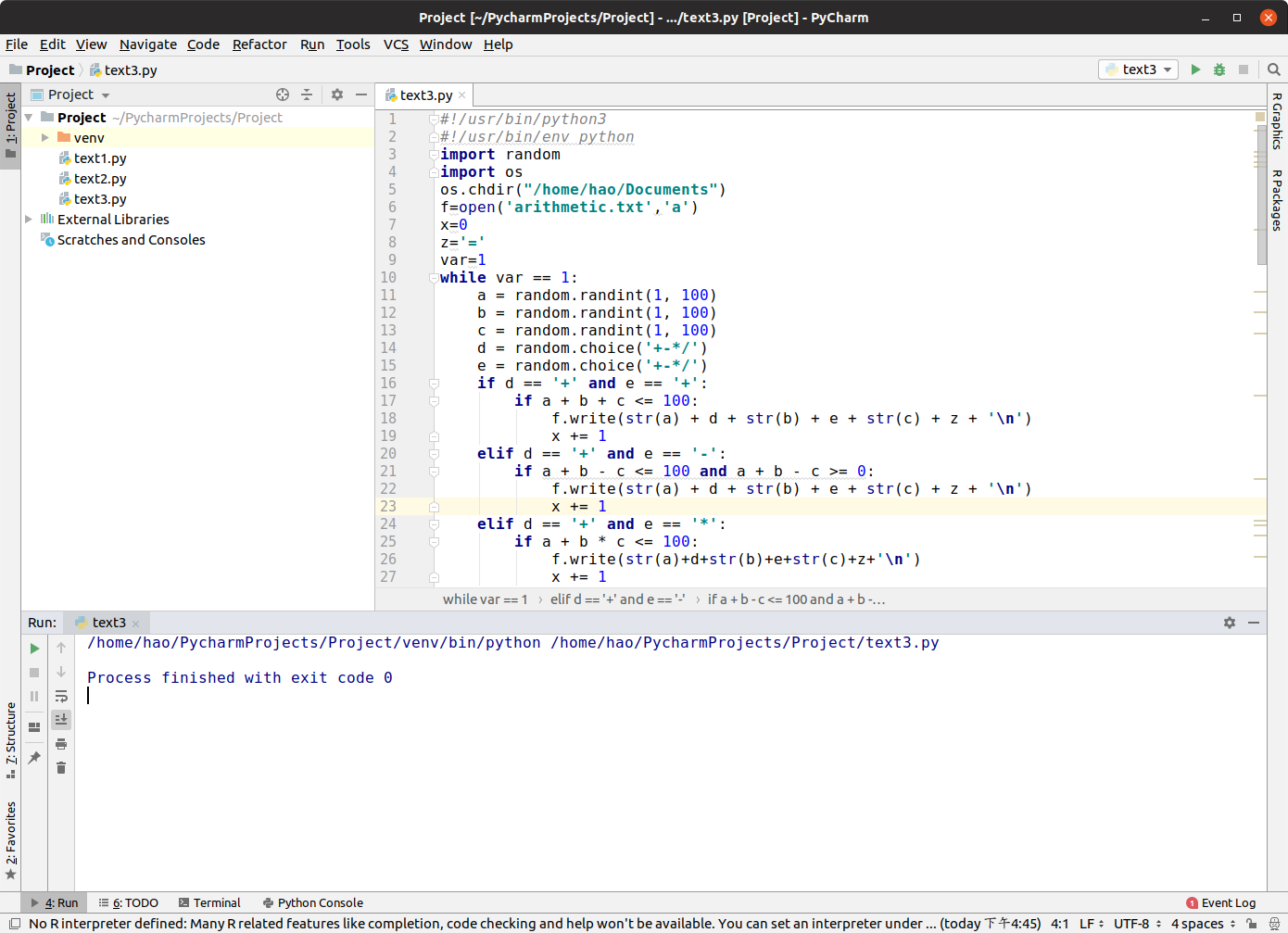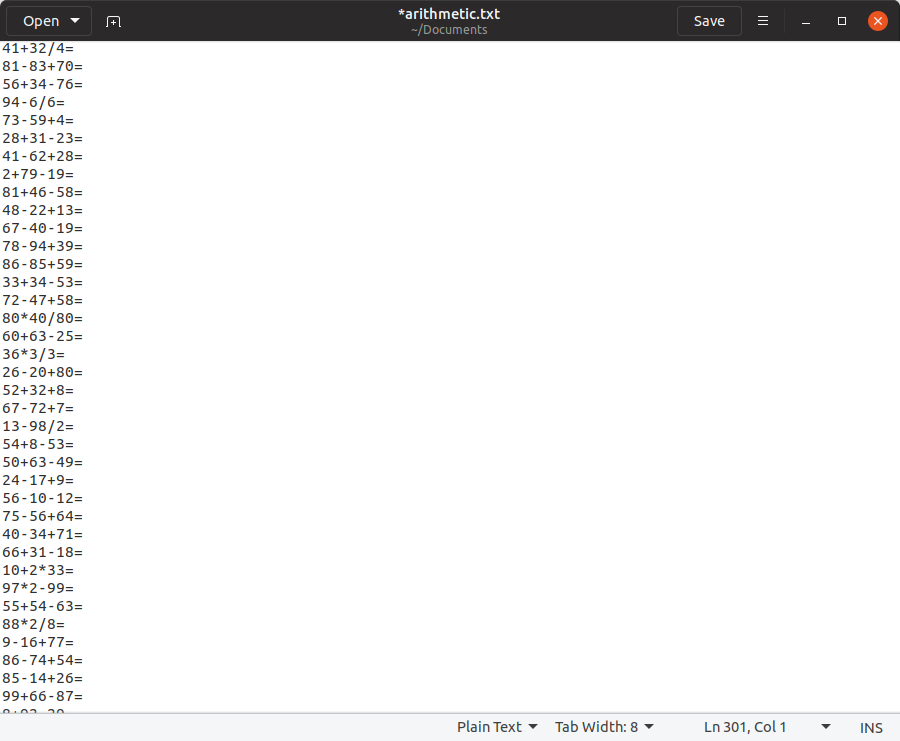# 软件工程综合实践-第二次作业-结对编程

1、互相鼓励，不容易心态爆炸

2、互相监督，不易偷懒

3、互相学习编程技巧，能力互补

4、多双眼睛，少点 bug  等等...

1、与合不来的人一起编程容易发生争执

2、一山不容二虎，开发者之间可能就某一问题发生分歧，造成不必要的内耗

3、开发人员可能会在工作时交谈一些与工作无关的事，分散注意力，造成效率低下  等等...

import random
import os
os.chdir("/home/hao/Documents")
f=open('arithmetic.txt','a')
x=0
z='='
var=1
while var == 1:
a = random.randint(1, 100)
b = random.randint(1, 100)
c = random.randint(1, 100)
d = random.choice('+-*/')
e = random.choice('+-*/')
if d == '+' and e == '+':
if a + b + c <= 100:
f.write(str(a) + d + str(b) + e + str(c) + z + '\n')
x += 1
elif d == '+' and e == '-':
if a + b - c <= 100 and a + b - c >= 0:
f.write(str(a) + d + str(b) + e + str(c) + z + '\n')
x += 1
elif d == '+' and e == '*':
if a + b * c <= 100:
f.write(str(a)+d+str(b)+e+str(c)+z+'\n')
x += 1
elif d == '+' and e == '/':
if b % c == 0 and a + b / c <= 100:
f.write(str(a) + d + str(b) + e + str(c) + z + '\n')
x += 1
elif d == '-' and e == '+':
if a - b + c <= 100 and a - b + c >= 0:
f.write(str(a) + d + str(b) + e + str(c) + z + '\n')
x += 1
elif d == '-' and e == '-':
if a - b - c <= 100 and a - b - c >= 0:
f.write(str(a) + d + str(b) + e + str(c) + z + '\n')
x += 1
elif d == '-' and e == '*':
if a - b * c <= 100 and a - b * c >= 0:
f.write(str(a) + d + str(b) + e + str(c) + z + '\n')
x += 1
elif d == '-' and e == '/':
if b % c == 0 and a - b / c <= 100:
f.write(str(a) + d + str(b) + e + str(c) + z + '\n')
x += 1
elif d == '*' and e == '+':
if a * b + c <= 100:
f.write(str(a) + d + str(b) + e + str(c) + z + '\n')
x += 1
elif d == '*' and e == '-':
if a * b - c <= 100 and a * b - c >= 0:
f.write(str(a) + d + str(b) + e + str(c) + z + '\n')
x += 1
elif d == '*' and e == '*':
if a * b * c <= 100:
f.write(str(a) + d + str(b) + e + str(c) + z + '\n')
x += 1
elif d == '*' and e == '/':
if (b % c == 0 or a % c == 0) and a * b / c <= 100:
f.write(str(a) + d + str(b) + e + str(c) + z + '\n')
x += 1
elif d == '/' and e == '+':
if a % b == 0 and a / b + c <= 100:
f.write(str(a) + d + str(b) + e + str(c) + z + '\n')
x += 1
elif d == '/' and e == '-':
if a % b == 0 and a / b - c <= 100 and a / b - c >= 0:
f.write(str(a) + d + str(b) + e + str(c) + z + '\n')
x += 1
elif d == '/' and e == '*':
if (a % b == 0 or (a * c) % b == 0) and a / b * c <= 100:
f.write(str(a) + d + str(b) + e + str(c) + z + '\n')
x += 1
elif d == '/' and e == '/':
if b!=c and b!=1 and c!=1:
if (a%b==0 and (a/b)%c==0) or (a%c==0 and (a/c)%b==0):
f.write(str(a) + d + str(b) + e + str(c) + z + '\n')
x+=1
if x == 300:
break
f.write('\n')
f.close()

（1）程序编译成功截图（2）在txt文件里的输出片段截图1、在开始的时候，我们在百度上搜索出来等号并不是运算符，出于严谨考虑，最后我们决定出的四则运算题是有三个数字和两个运算符（加减乘除）的混合运算题

2、通过多个随机函数来取多个不同的随机数避免了题目的重复

3、可定制：输出的题目都保存在了txt文件里，可以直接打印，且输出每三百题之间留有间隔

4、因为服务对象是小学生，所以我们确保出的每道题目中的除法都没有余数，同时也意味着不支持分数

5、加减时的最终结果一定大于等于0且小于等于100（中间结果可能大于100或小于0）

1、通过反复测试发现随机出的题中，有些种类混合运算题如连乘题（a*b*c）出现的概率过于低（因为结果要在100以内），暂时还无法平衡混合运算题中各种题目出现的概率

2、由于水平有限，我们并不能在题目里加上括号

3、可拓展性仍需商榷

（1）优点是：经过编程时的反复测试得出严谨性较高的判断条件，基本保证不会出现错题

（2）缺点是：判断条件太多显得整段程序比较low，可读性不高，计算量偏大，写完以后发现可以通过switch语句优化程序

（3）可扩展性：以后我们可以写一个客户端，让学生可以在客户端上做题并判断对错，且每个人做的题目都不会重复

通过这次作业，切实体验到了结对编程的过程，我们发现，在我们得出设计思路，并将它们初次转化成代码后，其实只完成了一部分。由于个人的疏忽，输入的错误，以及设计思路的偏差，往往会让我们的程序出现bug，修改bug会消耗我们大量的时间。而结对编程的好处就在于此，由于身边有个领航员角色的存在，在编写代码时，一旦出现输入错误，就会有人及时的提醒。不仅如此，在设计代码时，有个同伴可以一起讨论，融合两个人不同的见解和观点，我们往往可以得出更加准确且更加高效的设计思路。就我们本次实验的编程而言，有了领航员的存在，我们在完成代码后，几乎没有花什么时间在修改bug上，这提高了我们编程的效率，而且我们二人一起讨论出来的编程思路，也使得我们的代码功能更全面，更严谨。所以我们认为，结对编程的优势主要就在于设计思路的共享，还有及时修改自己在编程时犯下的小错误，这些错误在另一个人眼中会十分明显。而且两人轮流编程，不会太过疲惫，结对编程可以很大程度上提高编程效率，因此十分适合敏捷开发。如果未来我们从事软件开发的工作，我们会十分乐于进行结对编程，因为这会极大的改善我们的编程体验。

posted on 2019-03-20 00:08 豪文丶 阅读( ...) 评论( ...) 编辑 收藏

03-2549
03-18606
03-07158
09-29
03-25
09-10
08-03
08-03
08-08

### “相关推荐”对你有帮助么？

•非常没帮助
•没帮助
•一般
•有帮助
•非常有帮助被折叠的  条评论 为什么被折叠?到【灌水乐园】发言点击重新获取扫码支付1.余额是钱包充值的虚拟货币，按照1:1的比例进行支付金额的抵扣。
2.余额无法直接购买下载，可以购买VIP、C币套餐、付费专栏及课程。余额充值